Related Articles

# Class 8 RD Sharma Solutions – Chapter 17 Understanding Shapes Special Types Of Quadrilaterals – Exercise 17.3

• Last Updated : 12 Mar, 2021

### Question 1. Which of the following statements are true for a rectangle?

(i) It has two pairs of equal sides.

(ii) It has all its sides of equal length.

(iii) Its diagonals are equal.

(iv) Its diagonals bisect each other.

(v) Its diagonals are perpendicular.

(vi) Its diagonals are perpendicular and bisect each other.

(vii) Its diagonals are equal and bisect each other.

(viii) Its diagonals are equal and perpendicular and bisect each other.

(ix) All rectangles are squares.

(x) All rhombuses are parallelograms.

(xi) All squares are rhombuses and also rectangles.

(xii) All squares are not parallelograms.

Solution:

(i) It has two pairs of equal sides.

This statement is True.

(ii) It has all its sides of equal length.

This statement is False because only the pair of opposite sides are equal in a rectangle.

(iii) Its diagonals are equal.

This statement is True.

(iv) Its diagonals bisect each other.

This statement is True.

(v) Its diagonals are perpendicular.

This statement is False because diagonals are not perpendicular.

(vi) Its diagonals are perpendicular and bisect each other.

This statement is False because diagonals are not perpendicular, they only bisect each other.

(vii) Its diagonals are equal and bisect each other.

This statement is True.

(viii) Its diagonals are equal and perpendicular, and bisect each other.

This statement is False because the length of the diagonals are equal, and they bisect each other, but they are not perpendicular.

(ix) All rectangles are squares.

This statement is False because all the rectangles are not square.

(x) All rhombuses are parallelograms.

This statement is True.

(xi) All squares are rhombuses and also rectangles.

This statement is True.

(xii) All squares are not parallelograms.

This statement is False because the opposite sides are parallel and equal, so all squares are parallelograms.

### Question 2. Which of the following statements are true for a square?

(i) It is a rectangle.

(ii) It has all its sides of equal length.

(iii) Its diagonals bisect each other at right angle.

(v) Its diagonals are equal to its sides.

Solution:

(i) It is a rectangle.

This statement is True.

(ii) It has all its sides of equal length.

This statement is True.

(iii) Its diagonals bisect each other at right angle.

This statement is True.

(v) Its diagonals are equal to its sides.

This statement is False because the length of the diagonals are not equal to the length of the sides of the square.

### Question 3. Fill in the blanks in each of the following, so as to make the statement true :

(i) A rectangle is a parallelogram in which ________.

(ii) A square is a rhombus in which __________.

(iii) A square is a rectangle in which ___________.

Solution:

(i) A rectangle is a parallelogram in which one angle is a right angle.

(ii) A square is a rhombus in which one angle is a right angle.

(iii) A square is a rectangle in which adjacent sides are equal.

### Question 4. A window frame has one diagonal longer than the other. Is the window frame a rectangle? Why or why not?

Solution:

The window frame is not a rectangle because the length of the diagonals of a rectangle are equal.

### Question 5. In a rectangle ABCD, prove that ΔACB ≅ΔCAD.

Solution:

Draw a rectangle,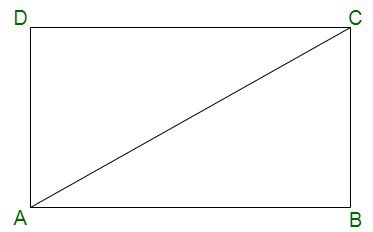Now in the given figure

Diagonal = AC

AB = CD            [Opposite sides of a rectangle are equal]

BC = DA

AC = CA           [Common Sides]

So, by SSS both the triangles are congruent

### Question 6. The sides of a rectangle are in the ratio 2 : 3, and its perimeter is 20 cm. Draw the rectangle.

Solution:

Given: Perimeter = 20cm

Ratio = 2:3

Let ABCD is a rectangle

So, let us assume ‘x’ be the side of rectangle

Length(l) = 3x

By using the formula, we get

Perimeter of the rectangle = 2(length + breadth)

20 = 2(3x + 2x)

10x = 20

x = 20/10 = 2

Hence, Length = 3×2 = 6cm and breadth = 2×2 = 4cm

So, now we draw a rectangle of length = 6cm and breadth = 4cm.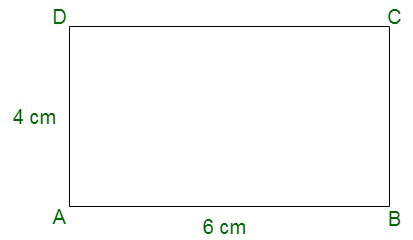### Question 7. The sides of a rectangle are in the ratio 4 : 5. Find its sides if the perimeter is 90 cm.

Solution:

Given: Perimeter = 90cm

Ratio = 4:5

Let ABCD is a rectangle

So, let us assume ‘x’ be the side of rectangle

Length (l) = 5x

By using the formula, we get

Perimeter of the rectangle = 2(length + breadth)

90 = 2(5x + 4x)

18x = 90

x = 90/18 = 5

Hence, Length = 5×5 = 25cm and Breadth = 4×5 = 20cm

So, now we draw a rectangle of length = 25cm and breadth = 20cm.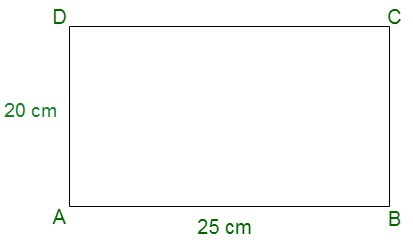### Question 8. Find the length of the diagonal of a rectangle whose sides are 12 cm and 5 cm.

Solution: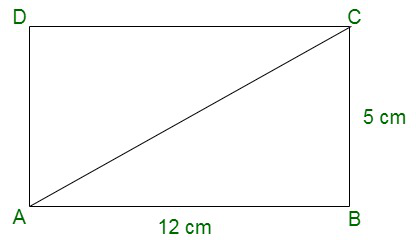Given that in rectangle ABCD,

AB = 12cm

BC = 5cm

Find: the length of AC

In ΔABC

By using Pythagoras theorem,

AC2 = AB2 + BC2

AC2 = 122 + 52

AC2 = 144 + 25

AC2 = 169

AC = √169

AC = 13cm

Hence, the length of the diagonal AC = 13cm.

### Question 9. Draw a rectangle whose one side measures 8 cm and the length of each of whose diagonals is 10 cm.

Solution:

Given that

One side of the rectangle = 8cm.

Diagonal length = 10cm

So, Now we are going to construct a rectangle using the following steps:

(i) Draw a line named as AB of length 8 cm.

(ii) Now, from point A create an arc of length 10 cm and mark it as a point C.

(iii) Now draw a 90° angle from point B and then join the arc from point A which cuts at point C.

(iv) Join lines AC and BC.

(v) Now draw a 90° angle from point A and cut an arc from C point of length 8 cm for D point

(vi) Now, join lines CD and AD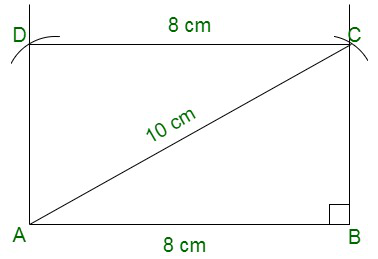### Question 10. Draw a square whose each side measures 4.8 cm.

Solution:

Given that

Side = 4.8cm.

So, Now we are going to construct a square using the following steps:

(i) Draw a line named as AB of length 4.8 cm.

(ii) Now draw perpendiculars(90° angle) from points A and B.

(iii) From point A and B create an arc of length 4.8 cm on the perpendiculars to get point D and C.

(iv) After creating arc join line DC, AD and BC.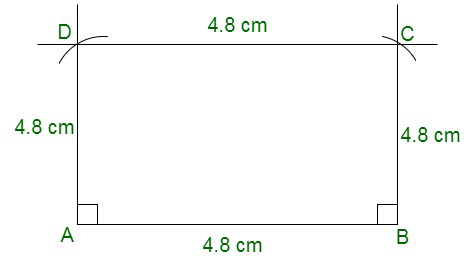### (ii) Four right angles

Solution:

(i) Square and Rhombus.

(ii) Square and Rectangle.

### (iv) A rectangle?

Solution:

(i) A square is a quadrilateral because it has all sides of equal length.

(ii) A square is a parallelogram because the opposite sides of a square are equal and parallel.

(iii) A square is a rhombus because all sides of a square are of equal length and the opposite sides are parallel.

(iv) A square is a rectangle because the opposite sides of a square are equal and each angle is of 90°.

### (iii) are equal.

Solution:

(i) Parallelogram, Rectangle, Rhombus and Square.

(ii) Rhombus and Square.

(iii) Square and Rectangle.

### Question 14. ABC is a right-angled triangle and O is the mid-point of the side opposite to the right angle. Explain why O is equidistant from A, B, and C.

Solution: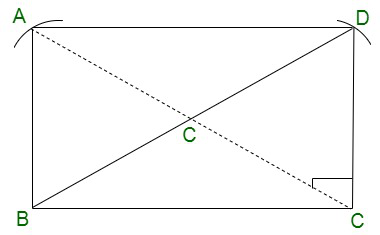Given that triangle ABC is a right-angled triangle, in which O is the midpoint of hypotenuse AC

So, OA = OC

Now, we draw CD||AB and join AD,

such that AB = CD and AD = BC.

So, the quadrilateral ABCD is a rectangle because each angle is a right

angle and opposite sides are equal and parallel.

So, AC = BD           [Length of the diagonals are equal]

AO = OC = BO = OD

Therefore, O is equidistant from A, B and C.

### Question 15. A mason has made a concrete slab. He needs it to be rectangular. In what different ways can he make sure that it is rectangular?

Solution:

The concrete slab can be a rectangle if each angles is 90° and the diagonals are equal.

Attention reader! Don’t stop learning now. Participate in the Scholorship Test for First-Step-to-DSA Course for Class 9 to 12 students.

My Personal Notes arrow_drop_up